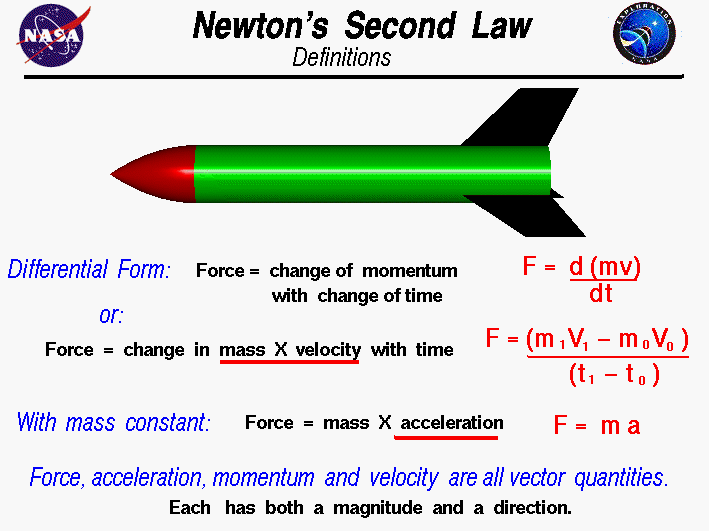+ Text Only Site
+ Non-Flash Version
+ Contact GlennSir Isaac Newton first presented his three laws of motion in the "Principia Mathematica Philosophiae Naturalis" in 1686. His second law defines a force to be equal to the differential change in momentum per unit time as described by the calculus of mathematics, which Newton also developed. The momentum is defined to be the mass of an object m times its velocity v. So the differential equation for force F is: F = d(m * v) / dt If we take very small time increments, we can write a difference equation from the differential equation: F = (m1 * v1 - m0 * v0) / (t1 - t0) If the mass is a constant, using the definition of acceleration a as the change in velocity with time, the second law reduces to the more familiar product of a mass and an acceleration: F = m * a The force, acceleration, velocity, and momentum have both a magnitude and a direction associated with them. Scientists and mathematicians call this a vector quantity. The equations shown here are actually vector equations and can be applied in each of the component directions. The external force F for a rocket is a combination of the weight, thrust, drag and lift of the vehicle. If we know the external force F, the equations can be solved to describe the motion of a rocket in flight. For some simple cases, we can write equations which describe the location and velocity of the rocket at any time in the flight. For the more general case, we can use a computer program to solve the equations. The assumption of constant mass works well for stomp rockets and fairly well for solid model rockets, but not very well for bottle rockets or full scale rockets because of the large decrease in the mass of these rockets during flight as the propellants are expelled. Guided ToursNewton's Laws of Motion:Rocket Translation:Forces, Torques and Motion:Flight Equations:Activities: Fundamental Terminology: Grade 10-12 Newton Car: Grade 10-12 Related Sites: Rocket Index Rocket Home Beginner's Guide Home+ Inspector General Hotline + Equal Employment Opportunity Data Posted Pursuant to the No Fear Act + Budgets, Strategic Plans and Accountability Reports + Freedom of Information Act + The President's Management Agenda + NASA Privacy Statement, Disclaimer, and Accessibility CertificationEditor: Tom Benson NASA Official: Tom Benson Last Updated: May 13 2021 + Contact Glenn